RGL
Realistic Graphics Lab

# Homework 4¶

### CS328 — Numerical Methods for Visual Computing and Machine Learning¶

Out on Thursday 18/11/2021, due on Thursday 02/12/2021

This notebook contains literate code, i.e. brief fragments of Python surrounded by descriptive text. Please complete/extend this notebook for your homework submission:

• Before handing in, please make sure that your notebook runs from top to bottom after selecting "Kernel->Restart & Run All" without causing any errors. To simplify the grading process, please do not clear the generated output.

Make sure to use the reference Python distribution so that project files can be opened by the TAs. In this course, we use Anaconda, specifically the version based on Python 3.8.

Please keep in mind that homework assignments must be done individually.

In this assignment, we will make use a library called bqplot developed by Bloomberg, which enables fully interactive plots within the Jupyter notebook. By default, this library is not installed on the Anaconda Python distribution we're using in this course, hence you will need to install it first. To do so, open the console (cmd.exe on Windows or the standard terminal on Linux or MacOS) and enter the following commands.

pip install bqplot
jupyter nbextension enable --py --sys-prefix bqplot
jupyter nbextension enable --py --sys-prefix widgetsnbextension

However, note that some systems like MacOS ship a with default version of Python that is very old and outdated. To ensure that the right versions of the pip and jupyter commands are executed, it's safer to first have to navigate to the directory where Anaconda was installed and then execute the commands there. The following screenshots show how to do this on the various supported platforms (note the ./ prefix on Linux and Mac OS).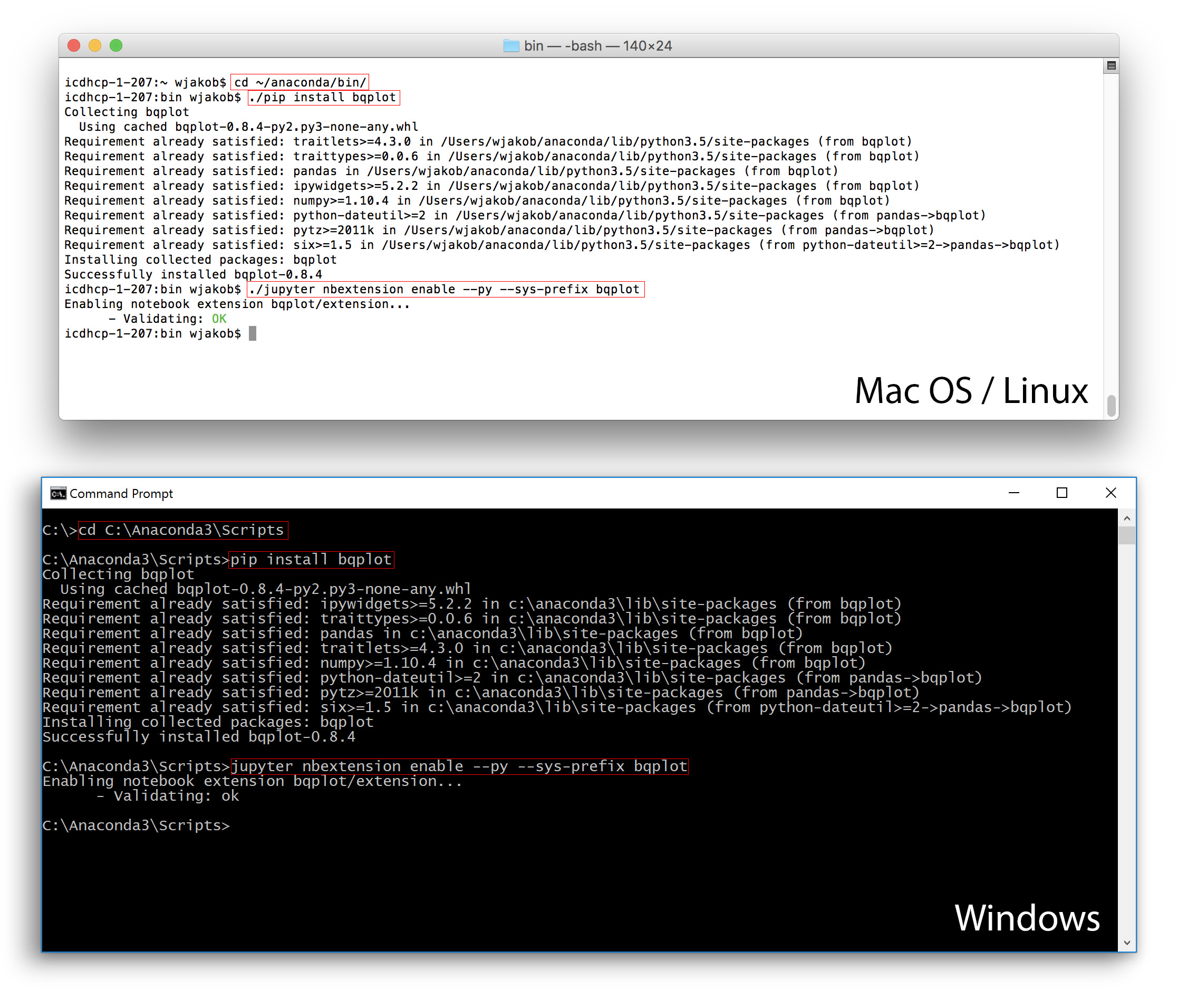Afterwards, you will have to restart Jupyter notebook for the change to become effective. You can enter and run the following commands in a new cell to check if bqplot was installed correctly—they should display a figure with a pie chart.

import bqplot as bqp
bqp.Figure(marks=[bqp.Pie(sizes=range(1, 6))], title='A pie chart!')


# Prelude¶

We begin by importing essential NumPy/SciPy/Matplotlib components that are needed to complete the exercises. The package scipy.optimize is new -- we'll use it for the last portion of this homework. The ipywidgets package is used internally by some of the code snippets provided by us.

In [ ]:
import numpy as np
import scipy.linalg as la

# New: Optimization package
import scipy.optimize as opt

# bqplot plotting library
import bqplot as bqp

# Import graphical user interface components used below
from ipywidgets import interact
from ipywidgets import FloatSlider, VBox


# Inverse Kinematics using Newton's method and the Pseudoinverse¶

$$\newcommand{\vp}{\mathbf{p}} \newcommand{\vx}{\mathbf{x}} \newcommand{\vf}{\mathbf{f}} \newcommand{\mA}{\mathbf{A}}$$

Lifelike computer-animated characters and animals are increasingly pervasive in today’s society: they are now commonly encountered in games, advertisements, feature animation, and a variety of other fields. It’s interesting to realize that all of these animated characters initially start out as static 3D shapes, not unlike stone sculptures that are unable to move. Before a character built in this way can be seen in motion, the precise way in which its shape can change over time must be characterized mathematically. The most common way of doing this entails designing a customized 3D skeleton that is then attached to its outer skin. Subsequently, any adjustment to the posture of the skeleton will result in a corresponding change to the posture of the character. The figure below shows a simple character with an embedded skeleton and two example poses that were created by rotating the joints of the skeleton.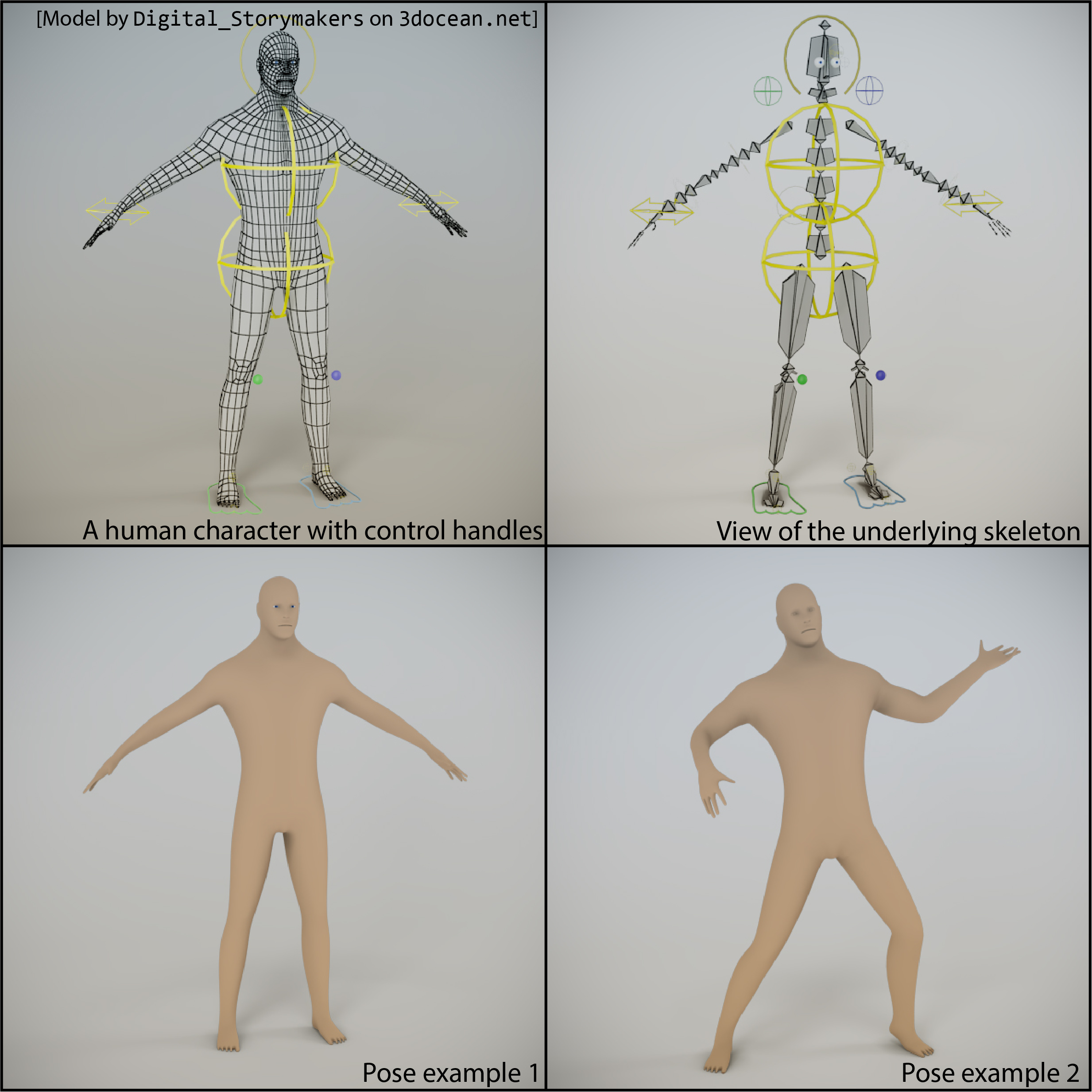A skeleton can range from a few elements to massively complex arrangements that reproduce the entire biological structure of the person or animal in question. In either case, the skeleton consists only of repeated instances of one basic component: a bone. Fortunately, computer graphics bones are much simpler than actual bones in real life.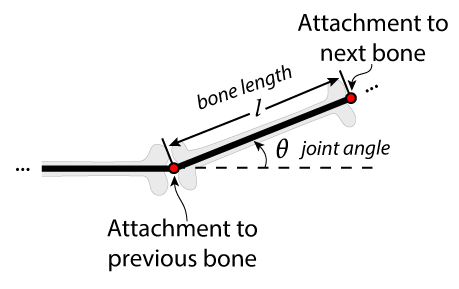As you can see in the above figure, each bone is essentially a line segment with a joint where it is connected to the previous bone. The bone can rotate in any way, but the connection between two bones can never move apart. Each bone also has a fixed length, in other words: it is rigid and never compresses or expands. On the other side, the next bone is generally attached. In 3D, a variety of rotations are possible (e.g. around the X, Y, or Z axis). In two dimensions things are simpler, and a single angle is enough to completely characterize the rotation of a bone relative to its predecessor bone. Everything in this homework will be in two dimensions to keep things simple.

## Part 1: Forward Kinematics (35 pts)¶

In this exercise, we'll investigate the mathematics of a very simple kind of "skeleton": a chain of bones in two dimensions with joint positions $\vp_i = (x_i, y_i)$. The first joint is rigidly attached to the origin (i.e. $\vp_0 = (0, 0)$) while the other joints and bones are free to move in any way. For simplicitly, we'll also assume that all of the bones have the same length $l_1=l_2=\ldots=1$.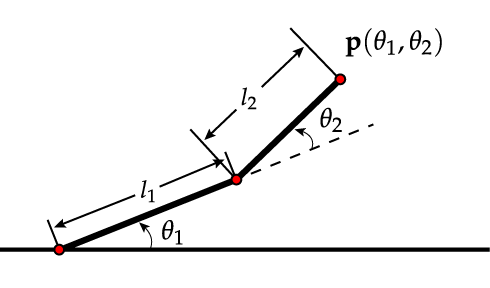Each parameter $\theta_i\in[0,2\pi]$ specifies the counter-clockwise angle that the associated bone from joint $\vp_{i-1}$ to joint $\vp_i$ makes with its predecessor bone (the pair of bones are parallel if $\theta_i=0$). The first bone doesn't have a predecessor, hence $\theta_1$ is measured relative to the $X$ axis. Note how the complete set of bone angles $\theta_1, \theta_2, \ldots$ is all the information we need to compute the precise positions of all the joint positions in Euclidean space.

Forward kinematics (FK) is defined as the problem of converting a set of bone angles $\theta_i$ into joint positions $\vp_i$. Since $\vp_i$ depends on all of the preceding angles, we can think of each joint position as a function $\vp_i=\vp(\theta_1,\ldots,\theta_{i})$

TODO (15 pts): Your first task is to create a function chain_simple, which solves the forward kinematics for a chain with at most one bone. The function should take an array of angles as a parameter, which can be of length 0 or 1 (use the Python len() function to query the length of an array). When no angles are specified, the function should return the position of the first joint $(x_0,y_0)=(0, 0)$ as an 1D NumPy array. When a single angle is specified, it should return the position $x_1, y_1$.

Ensure that your implementation returns the expected example values in the cell below (up to minor rounding errors).

In [ ]:
# TODO
def chain_simple(theta):
return np.array([]) # Replace!

# TESTS (Do not modify!)
print('chain_simple:', chain_simple([]))
print('reference:   ', np.array([0.,0.]))
print()
print('chain_simple:', chain_simple([0.]))
print('reference:   ', np.array([1., 0.]))
print()
print('chain_simple:', chain_simple([np.pi / 4]))
print('reference:   ', np.array([ 0.70710678,  0.70710678]))


### 1.1 Helper function¶

We provide the function fk_demo() below to interactively explore the possible chain configurations via forward kinematics. The implementation uses the bqplot library mentioned above and is fairly technical. You are welcomed but not expected to read or understand how it works.

In [ ]:
def fk_demo(chain_func, theta, extra = [[],[]]):
'''
This function visualizes the configuration of a chain of bones
and permits interactive changes to its state. It expects two arguments:

chain_func: a function that implements forward kinematics by
turning a sequence of angles (theta_1, theta_2, ..., theta_n) into
the position of the last joint of this chain (x_n, y_n).

theta: an array with the initial angles of all joints

extra: An optional argument which can be used to plot
additional points that are highlighted in red
'''

# Function which repeatedly calls chain_func to compute all joint positions
def chain_all(theta):
return np.column_stack([chain_func(theta[:i]) for i in range(0, len(theta) + 1)])

# Determine size and initial configuration
size = len(theta)
positions = chain_all(theta)

# Define the range of the plotting frame
scales = { 'x': bqp.LinearScale(min=-size-1, max=size+1),
'y': bqp.LinearScale(min=-size-1, max=size+1) }

# Create a scatter plot (for joints), a line plot (for bones), and
# another scatter plot (to draw extra points specified the extra argument)
scat  = bqp.Scatter(scales=scales)
lines = bqp.Lines(scales=scales)
scat2 = bqp.Scatter(scales=scales, colors=['red'])

# Create a figure that combines the three plots
figure = bqp.Figure(marks=[scat, scat2, lines])
figure.layout.height = '500px'
figure.layout.width = '500px'

# Initialize the plots with the initial data
scat.x, scat.y = positions
lines.x, lines.y = positions
scat2.x, scat2.y = extra

sliders = []

# For each angle theta_i,
for i in range(len(theta)):
# Create a graphical slider
slider = FloatSlider(min=0, max=2*np.pi, value=theta[i], step=1e-3)

# Define a callback function that will be triggered when the slider is moved
def callback(value, i = i):
theta[i] = value['new']
positions = chain_all(theta)
scat.x, scat.y = positions
lines.x, lines.y = positions

# "Attach" the callback function to the slider
slider.observe(callback, 'value')
sliders.append(slider)

# Combine the plots and sliders in a vertical arrangement
return VBox([*sliders, figure])


### 1.2 Visualization of the forward kinematics¶

TODO (0pts): To ensure that your implementation of chain_simple satisfies all the specifications, run the following cell that invokes the fk_demo() function with arguments chain_simple and [0.] (the initial parameters of a flat chain). You should be able to drag a slider from 0 to $2\pi$ and see a visual representation of a 1-bone chain turning counter-clockwise.

In [ ]:
fk_demo(chain_simple, )


### 1.3 Longer chains¶

TODO (15 pts): Create a function chain, which solves the forward kinematics for an arbitrarily long sequence of bones. The function should take an arbitrary-length array of angles as a parameter. When no angles are specified, the function should return the position $(x_0, y_0)$ as before. When $i$ angles are specified, it should (only) return the joint position $(x_{i}, y_{i})$.

Ensure that your implementation returns the expected example values in the cell below (up to minor rounding errors).

In [ ]:
# TODO
def chain(thetas):
return np.array([]) # Replace!

# TESTS (Do not modify!)
print('chain:     ', chain([0.1, 0.2, 0.3, 0.4]))
print('reference: ', np.array([ 3.31597858,  1.80146708]))
print()
print('chain:     ', chain([np.pi, np.pi, np.pi, np.pi]))
print('reference: ', np.array([0, 0]))


### 1.4 Attempting to reach a certain position¶

Run the command fk_demo(chain, [0, 0, 0, 0, 0], [[-2], ]) below. You should see a chain with five segments and five corresponding sliders, as well as an additional point highlighted in red.

TODO (5 points): Find a configuration of angles that brings the endpoint of the chain as close as possible to the highlighted location [-2, 3]]. An exact match is not necessary, but the points should overlap by a significant margin. Copy the parameters you found into the argment list of the fk_demo function call.

In [ ]:
# TODO


## Part 2: Inverse Kinematics (50 pts)¶

Problems similar to the one in Section 1.4 are tedious to solve by hand: all of the parameters are interdependent and must be adjusted in a coordinated manner. So-called inverse kinematics techniques apply numerical root finding to determine solutions to this problem in an automated way. Most modern animation systems have builtin support for inverse kinematics since it allows for a much more convenient workflow: rather than having to tweak each individual bone, artists can directly specify a target shape, and the system will automatically infer all the necessary rotations.

In this part of the exercise, we will use inverse kinematics to automatically determine $\theta_1,\ldots,\theta_n$ such that

$$\vp(\theta_1,\ldots,\theta_n) = \vp_{\mathrm{target}}$$

for a given value $\vp_{\mathrm{target}}\in\mathbb{R}^2$. In other words: the user can move around the endpoint of the chain, and the skeleton will automatically reconfigure itself to follow. This is illustrated in the following figure: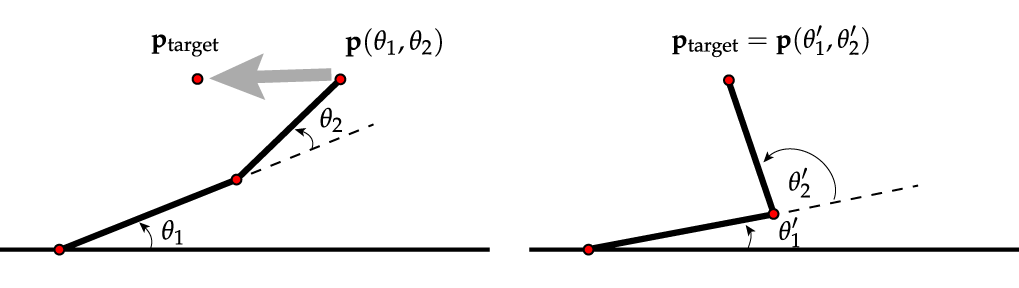All good numerical root finding techniques require the ability to evaluate the Jacobian of $\vp$, i.e. all the partial derivatives $\frac{\partial\vp(\theta_1,\ldots,\theta_n)}{\partial \theta_j}$. The partial derivatives encode how a small perturbation of each of the angles $\theta_j$ leads to a corresponding change in $\vp(\theta_1,\ldots,\theta_n)$. As before, we'll first look at a 1-segment chain and then derive a solution for the general problem.

TODO (10 pts): Implement a function dchain_simple(theta) which takes an array with one entry, and which computes the function $\frac{\partial \vp(\theta_1)}{\partial \theta_1}$. The return value should be a two-dimensional array with one column and two rows containing the partial derivatives of the coordinate values $x_1$ and $y_1$. You should use analytic methods -- approximating the derivatives via finite differences is not allowed.

Ensure that your implementation returns the expected example values in the cell below (up to minor rounding errors).

In [ ]:
# TODO
def dchain_simple(theta):
return np.array([]) # Replace!

# TESTS (Do not modify!)
print('dchain_simple: \n', dchain_simple())
print('reference:     \n', np.array([[0.], [1.]]))
print()
print('dchain_simple: \n', dchain_simple([np.pi / 4]))
print('reference:     \n', np.array([[-0.70710678], [ 0.70710678]]))


### 2.1 Implementing the full Jacobian function¶

Having finished the version for a single bone, we'll now turn to the full Jacobian $\nabla \vp(\theta_1, \ldots, \theta_n)$, which is a $2\times n$ matrix containing the partial derivatives with respect to all angles. You'll likely want to use a vector version of the product or chain rule in your implementation. Specifically, note that

$$\frac{\partial}{\partial t} \left[\mA(t)\vx(t)\right] = \mA'(t)\vx(t) + \mA(t)\vx'(t)$$

where $\mA(t)$ and $\vx(t)$ are a matrix and a vector depending on a parameter $t$, respectively.

TODO (25 pts): Implement a function dchain(theta) which accepts an 1D array of angles with length $\ge 1$ and computes the Jacobian $\nabla \vp(\theta_1, \ldots, \theta_n)$, a $2\times n$ matrix. Don't forget to shortly explain how your implementation was derived and why it works.

Ensure that your implementation returns the expected example values in the cell below (up to minor rounding errors).

In [ ]:
# TODO
def dchain(thetas):
return np.array([]) # Replace!

# TESTS (Do not modify!)
print('dchain: \n', dchain([0, 0, 0, 0]))
print('reference: \n', np.array([[ 0.,  0.,  0.,  0.], [ 4.,  3.,  2.,  1.]]))
print()
print('dchain: \n', dchain([0.1, 0.2, 0.3]))
print('reference: \n', np.array([[-0.9599961 , -0.86016268, -0.56464247], [ 2.77567627,  1.7806721 ,  0.82533561]]))


### 2.2 Solving the inverse kinematics problem using Newton's Method¶

Newton's method is one of the most widely used methods for finding solutions to systems of non-linear equations. It converges at a remarkable speed when started sufficiently close to a root, though there is generally no strict guarantee of convergence.

Given a function $\vf(\vx)$, Newton's method tries to find a solution to the equation $\vf = 0$ using steps of the form

$$\vx_{i+1}=\vx_i - \left(\nabla \vf\right)^{-1}\vf(\vx_{i}).$$

In the context of inverse kinematics, we want to apply Newton's method to solve an equation of the form

$$\vp(\theta_1,\ldots,\theta_n) = \vp_{\mathrm{target}}.$$

for a given reference position $\vp_{\mathrm{target}}\in\mathbb{R}^2$.

In other words: the unknowns are the angles $\theta_1,\ldots,\theta_n$, and the function whose root we seek maps to a two-dimensional domain. It is not immediately obvious how to apply Newton's method, since the Jacobian of the function has the shape $2\times n$ and hence cannot be inverted using standard techniques like the LU decomposition.

This should not be surprising. It is a consequence of the fact that many different configurations can be used to reach the same $\vp_{\mathrm{target}}$, which you may have noticed in part 1.4.

Fortunately, we can use the pseudoinverse, a generalization of the inverse to non-square matrices. In this specific case, the Jacobian is wide (i.e. it has more columns than rows), in which case the pseudoinverse will find the solution to a linear system which has the smallest $\|\cdot\|_2$-norm. That is excellent news, since it causes the IK solver to make small adjustments to the angles to reach a new position.

TODO (15 pts): Implement a function newton(theta, target) that takes a 1-dimensional array of angles as a starting guess as well as a 2D target position (also specified as a 1-dimensional array) as input. The implementation should perform a fixed 8 iterations of Newton's method to try to solve the equation $\vp(\theta_1,\ldots,\theta_n) = \vp_{\mathrm{target}}$ and return the final set of parameters $\theta_1,\ldots,\theta_n$ as an 1-dimensional NumPy array. You can use the function la.pinv to compute the pseudoinverse.

Ensure that your implementation is able to converge to the following positions (up to minor rounding errors)

1. Moving a 1-element chain from the default configuration to position $(0, 1)$, i.e. chain(newton(np.array([0.]), np.array([0., 1.]))) == np.array([0, 1])

2. Moving a 2-element chain from the default configuration to position $(0.5, 0.5)$, i.e. chain(newton(np.array([0., 0.]), np.array([0.5, 0.5]))) == np.array([0.5, 0.5])

In [ ]:
# TODO
def newton(thetas, target):
return np.array([]) # Replace!

# TESTS (Do not modify!)
# Moving a 1-element chain from the default configuration to position (0, 1)
print('chain(newton): ', chain(newton(np.array([0.]), np.array([0., 1.]))))
print('reference:     ', np.array([0, 1]))
print()
# Moving a 2-element chain from the default configuration to position (0.5, 0.5)
print('chain(newton): ', chain(newton(np.array([0., 0.]), np.array([0.5, 0.5]))))
print('reference:     ', np.array([0.5, 0.5]))


### 2.3 One more helper function¶

We provide the function ik_demo() below to interactively explore the possible chain configurations via inverse kinematics. Similar to fk_demo(), the function is fairly technical. You are welcomed but not expected to read or understand how it works.

In [ ]:
def ik_demo(solver, size):
theta = np.zeros(size, dtype=np.float64)

# Function which repeatedly calls chain to compute all joint positions
def chain_all(theta):
return np.column_stack([chain(theta[:i]) for i in range(0, len(theta) + 1)])

# Callback that is invoked when the user drags the red endpoint around
def refresh(_):
# 'theta' is a variable of the parent function, we want to modify it here
nonlocal theta

# Target position
target = np.array([scat2.x, scat2.y])

# Don't try to solve the problem if the user dragged the point out of the circle
if la.norm(target) > size:
return

# Call the provided IK solver
theta = solver(theta, target)

# Update the positions
values = chain_all(theta)
scat.x, scat.y = values
lines.x, lines.y = values

# Similar to fk_solver(), create a number of plots and merge them
scales = { 'x': bqp.LinearScale(min=-size-1, max=size+1),
'y': bqp.LinearScale(min=-size-1, max=size+1) }

scat  = bqp.Scatter(scales=scales)
lines = bqp.Lines(scales=scales)

# Create a circle which marks the boundary of where the red point can be moved
circle_x = np.cos(np.linspace(0, 2*np.pi, 100)) * size
circle_y = np.sin(np.linspace(0, 2*np.pi, 100)) * size
circle = bqp.Lines(x=circle_x, y=circle_y,
scales=scales, colors=['gray'])

# Special plot, which contains the red endpoint that can be moved
scat2 = bqp.Scatter(scales=scales,
enable_move=True,
update_on_move=True,
colors=['red'])

# Initialize the visualizations with the default configuration
values = chain_all(theta)
scat.x, scat.y = values
lines.x, lines.y = values
scat2.x, scat2.y = chain(theta).reshape(2, 1)

# Call the 'refresh' function when the red dot is moved
scat2.observe(refresh, names=['x', 'y'])

figure = bqp.Figure(marks=[scat, scat2, lines, circle])
figure.layout.height = '500px'
figure.layout.width = '500px'
return figure


### 2.4 Putting everything together¶

Finally, let's visualize the behavior of the completed inverse kinematics solver.

TODO :

1. Invoke the IK demonstration with 4 segments, i.e. ik_demo(newton, 4). You should be able to move the red endpoint with your mouse cursor, leading to a smooth adjustment of the chain configuration.

2. Invoke the IK demonstration with 30 segments, i.e. ik_demo(newton, 30).

In [ ]:
# TODO

In [ ]:
# TODO


## 3 Regularized Inverse Kinematics (15 points)¶

The inverse kinematics solution from Example 2 provides generally reasonable results by attempting to make the smallest change to the bone angles that will allow the chain to reach a particular point. However, in some cases there might be additional requirements we'd like the solution to satisfy. For instance, it might be unnatural for the character to bend a joint more than a few degrees. In this case, we could try to find a solution to an optimization problem that compromises between reaching the target position and bending joints by an overly large amount.

$$\DeclareMathOperator*{\argmin}{argmin} \argmin_{\theta_1,\ldots,\theta_n} \alpha\cdot\|\vp(\theta_1,\ldots,\theta_n)-\vp_\mathrm{target}\|_2^2 + \sum_{i=1}^n\theta_i^2$$

TODO: Optimize the above objective using the function opt.minimize and run your algorithm on a chain of length 5 (using the ik_demo function). Use $\alpha=10$ in the above formula (i.e. reaching the target point is quite important).

In [ ]:
# TODO


TODO: Now try to optimize the following function instead, using the same value of $\alpha$. What behavior do you observe? Note that the optimization seems to run much slower than in the previous case. Why do you think that is?

$$\DeclareMathOperator*{\argmin}{argmin} \argmin_{\theta_1,\ldots,\theta_n} \alpha\cdot\|\vp(\theta_1,\ldots,\theta_n)-\vp_\mathrm{target}\|_2^2 + \sum_{i=1}^n\left|\theta_i\right|$$
In [ ]:
# TODO